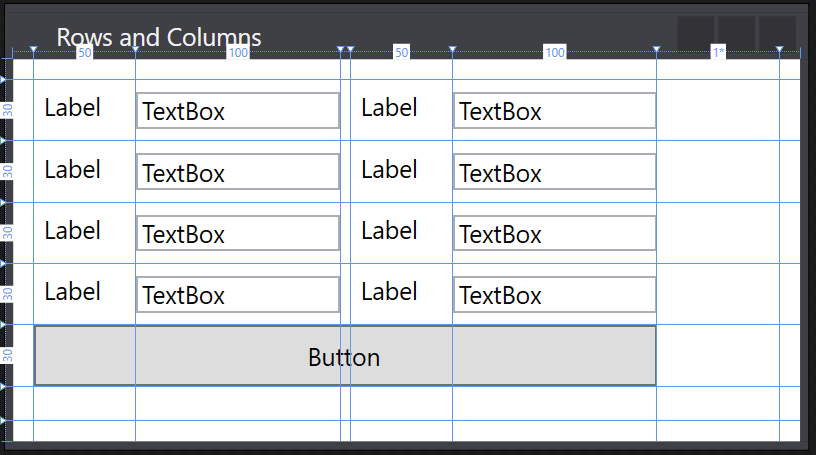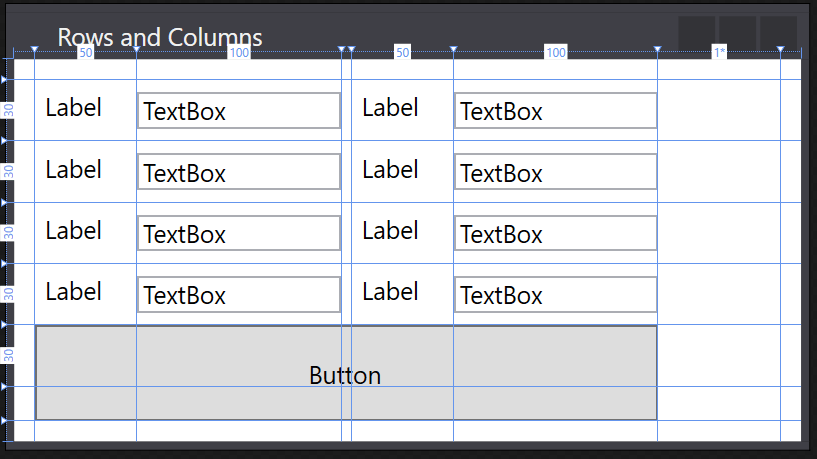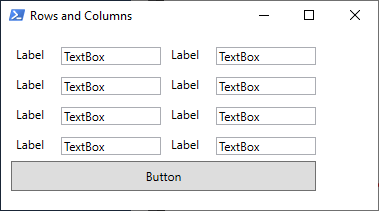## Creating Graphical User Interfaces – Working with rows and columns

This post was originally posted on my old blog: http://geekswithblogs.net/marcde/archive/2019/02/19/powershell-series-creating-graphical-user-interfaces–working-with-rows.aspx on February 19th, 2019

Rows and columns are things I’ve recently started to dive in to since I was getting fairly annoyed when I was building larger user interfaces. They’re pretty powerfull, but it took me a while to get my head around the capabilities… In essence you are setting up your grid element(s) to have predefined sizes, and drop working with the margin properties so whatever control you place in a row or column takes it up completely.

Also, it prevents your controls from changing position and size when you’re resizing a window, as well as making alignment of controls easier. Pretty neat!

## In the beginning…

So let’s say we’ve built a nice little basic GUI to show some information.Represented by the following XAML code:

```<Window
xmlns="http://schemas.microsoft.com/winfx/2006/xaml/presentation"
xmlns:x="http://schemas.microsoft.com/winfx/2006/xaml"
x:Name="MainWindow"
Title="Rows and Columns"
Height="217.893"
Width="392.894"
>

<!-- Grid area -->
<Grid Margin="0,0,0,0">

<Label x:Name="lblLabel" Content="Label" HorizontalAlignment="Left" Margin="10,10,0,0" VerticalAlignment="Top" Width="52"/>
<TextBox x:Name="txtTextBox" HorizontalAlignment="Left" Height="20" Margin="67,14,0,0" TextWrapping="Wrap" Text="TextBox" VerticalAlignment="Top" Width="120"/>
<Label x:Name="lblLabel0" Content="Label" HorizontalAlignment="Left" Margin="10,36,0,0" VerticalAlignment="Top" Width="52"/>
<Label x:Name="lblLabel1" Content="Label" HorizontalAlignment="Left" Margin="10,62,0,0" VerticalAlignment="Top" Width="52"/>
<Label x:Name="lblLabel2" Content="Label" HorizontalAlignment="Left" Margin="10,88,0,0" VerticalAlignment="Top" Width="52"/>
<TextBox x:Name="txtTextBox0" HorizontalAlignment="Left" Height="20" Margin="67,39,0,0" TextWrapping="Wrap" Text="TextBox" VerticalAlignment="Top" Width="120"/>
<TextBox x:Name="txtTextBox1" HorizontalAlignment="Left" Height="20" Margin="67,64,0,0" TextWrapping="Wrap" Text="TextBox" VerticalAlignment="Top" Width="120"/>
<TextBox x:Name="txtTextBox2" HorizontalAlignment="Left" Height="20" Margin="67,88,0,0" TextWrapping="Wrap" Text="TextBox" VerticalAlignment="Top" Width="120"/>
<Label x:Name="lblLabel3" Content="Label" HorizontalAlignment="Left" Margin="202,10,0,0" VerticalAlignment="Top" Width="52"/>
<Label x:Name="lblLabel4" Content="Label" HorizontalAlignment="Left" Margin="202,35,0,0" VerticalAlignment="Top" Width="52"/>
<Label x:Name="lblLabel5" Content="Label" HorizontalAlignment="Left" Margin="202,64,0,0" VerticalAlignment="Top" Width="52"/>
<Label x:Name="lblLabel6" Content="Label" HorizontalAlignment="Left" Margin="202,88,0,0" VerticalAlignment="Top" Width="52"/>
<TextBox x:Name="txtTextBox3" HorizontalAlignment="Left" Height="20" Margin="254,14,0,0" TextWrapping="Wrap" Text="TextBox" VerticalAlignment="Top" Width="120"/>
<TextBox x:Name="txtTextBox4" HorizontalAlignment="Left" Height="20" Margin="254,40,0,0" TextWrapping="Wrap" Text="TextBox" VerticalAlignment="Top" Width="120"/>
<TextBox x:Name="txtTextBox5" HorizontalAlignment="Left" Height="20" Margin="254,66,0,0" TextWrapping="Wrap" Text="TextBox" VerticalAlignment="Top" Width="120"/>
<TextBox x:Name="txtTextBox6" HorizontalAlignment="Left" Height="20" Margin="254,92,0,0" TextWrapping="Wrap" Text="TextBox" VerticalAlignment="Top" Width="120"/>
</Grid>
</Window>```

## Creating columns and rows

It’s fairly easy to add in Columns and Rows in to your GUI, it’s as simple as adding a few tags right under the <Grid> tag:

```<Grid>
<Grid.ColumnDefinitions>
</Grid.ColumnDefinitions>
<Grid.RowDefinitions>
</Grid.RowDefinitions>
</Grid>```

But this obviously doesn’t create a row or column! It merely sets up the scaffolding for us to start defining them… In order to add a column or row we need to use the <RowDefinition Height=””/> or <ColumnDefinition Width=””/> tag.

In our case I want to have an edge of 10 pixels wide around our borders which would mean my top and bottom rows need a height of 10, and the left and right columns need a width of 10. For the labels I will use a width of 100 pixels, whilst the textboxes columns will get a width of 150. Since I don’t want the labels on the right side to but up to the textboxes of the left side I will put in a column to separate them.

That would mean our grid code would look like this:

```<Grid Margin="0,0,0,0">
<Grid.ColumnDefinitions>
<ColumnDefinition Width="10"/>
<ColumnDefinition Width="50"/>
<ColumnDefinition Width="100"/>
<ColumnDefinition Width="5"/>
<ColumnDefinition Width="50"/>
<ColumnDefinition Width="100"/>
<ColumnDefinition Width="*"/>
<ColumnDefinition Width="10"/>
</Grid.ColumnDefinitions>
<Grid.RowDefinitions>
<RowDefinition Height="10"/>
<RowDefinition Height="30"/>
<RowDefinition Height="30"/>
<RowDefinition Height="30"/>
<RowDefinition Height="30"/>
<RowDefinition Height="30"/>
<RowDefinition Height="*"/>
<RowDefinition Height="10"/>
</Grid.RowDefinitions>
</Grid>```

Which looks like this in the GUI:You’ve probably noticed I created a row and a column where the height and width property are set to “*”. I call them filler rows/columns, since they will take up all the space that’s still available in your window size.

## Hey! Where did my controls go?!

Oh you noticed? Good! By default, all controls seem to live in Row 0, Column 0. So if you create columns and rows, your controls will still be there, you just won’t be able to see them… But let’s get that fixed now! Let’s start with our very first label:

`<Label x:Name="lblLabel" Content="Label" HorizontalAlignment="Left" Margin="10,10,0,0" VerticalAlignment="Top" Width="52"/>`

There’s a few properties we’ll need to remove to meet our criteria of filling the space, and a few to add so it get’s bound to a row and column

`<Label x:Name="lblLabel" Grid.Row="1" Grid.Column="1" Content="Label" HorizontalAlignment="Stretch" VerticalAlignment="Stretch" />`

As you can see, I’ve added 2 tags: Grid.Row and Grid.Column. Both of these place your control in the respective values (and they start counting from 0!). I’ve also set the HorizontalAlignment and VerticalAlignment properties to stretch, so the label would take up all the space available, and removed my margin and width properties, since they’re no longer needed… Rinse and repeat for all controls and you’re GUI will end up looking like this:And the code:

```<Window
xmlns="http://schemas.microsoft.com/winfx/2006/xaml/presentation"
xmlns:x="http://schemas.microsoft.com/winfx/2006/xaml"
x:Name="MainWindow"
Title="Rows and Columns"
Height="217.893"
Width="392.894"
>

<!-- Grid area -->
<Grid Margin="0,0,0,0">
<Grid.ColumnDefinitions>
<ColumnDefinition Width="10"/>
<ColumnDefinition Width="50"/>
<ColumnDefinition Width="100"/>
<ColumnDefinition Width="5"/>
<ColumnDefinition Width="50"/>
<ColumnDefinition Width="100"/>
<ColumnDefinition Width="*"/>
<ColumnDefinition Width="10"/>
</Grid.ColumnDefinitions>
<Grid.RowDefinitions>
<RowDefinition Height="10"/>
<RowDefinition Height="30"/>
<RowDefinition Height="30"/>
<RowDefinition Height="30"/>
<RowDefinition Height="30"/>
<RowDefinition Height="30"/>
<RowDefinition Height="*"/>
<RowDefinition Height="10"/>
</Grid.RowDefinitions>
<!-- A Label -->
<Label x:Name="lblLabel" Grid.Row="1" Grid.Column="1" Content="Label" HorizontalAlignment="Stretch" VerticalAlignment="Stretch" />
<TextBox x:Name="txtTextBox" Grid.Row="1" Grid.Column="2" HorizontalAlignment="stretch" TextWrapping="Wrap" Text="TextBox" VerticalAlignment="Center" />
<Label x:Name="lblLabel0" Grid.Row="2" Grid.Column="1" Content="Label" HorizontalAlignment="Stretch" VerticalAlignment="Stretch" />
<Label x:Name="lblLabel1" Grid.Row="3" Grid.Column="1" Content="Label" HorizontalAlignment="Stretch" VerticalAlignment="Stretch" />
<Label x:Name="lblLabel2" Grid.Row="4" Grid.Column="1" Content="Label" HorizontalAlignment="Stretch" VerticalAlignment="Stretch" />
<TextBox x:Name="txtTextBox0" Grid.Row="2" Grid.Column="2" HorizontalAlignment="Stretch" TextWrapping="Wrap" Text="TextBox" VerticalAlignment="Center" />
<TextBox x:Name="txtTextBox1" Grid.Row="3" Grid.Column="2" HorizontalAlignment="Stretch" TextWrapping="Wrap" Text="TextBox" VerticalAlignment="Center" />
<TextBox x:Name="txtTextBox2" Grid.Row="4" Grid.Column="2" HorizontalAlignment="Stretch" TextWrapping="Wrap" Text="TextBox" VerticalAlignment="Center" />
<Label x:Name="lblLabel3" Grid.Row="1" Grid.Column="4" Content="Label" HorizontalAlignment="Stretch" VerticalAlignment="Stretch" />
<Label x:Name="lblLabel4" Grid.Row="2" Grid.Column="4" Content="Label" HorizontalAlignment="Stretch" VerticalAlignment="Stretch" />
<Label x:Name="lblLabel5" Grid.Row="3" Grid.Column="4" Content="Label" HorizontalAlignment="Stretch" VerticalAlignment="Stretch" />
<Label x:Name="lblLabel6" Grid.Row="4" Grid.Column="4" Content="Label" HorizontalAlignment="Stretch" VerticalAlignment="Stretch" />
<TextBox x:Name="txtTextBox3" Grid.Row="1" Grid.Column="5" HorizontalAlignment="Stretch" TextWrapping="Wrap" Text="TextBox" VerticalAlignment="Center" />
<TextBox x:Name="txtTextBox4" Grid.Row="2" Grid.Column="5" HorizontalAlignment="Stretch" TextWrapping="Wrap" Text="TextBox" VerticalAlignment="Center" />
<TextBox x:Name="txtTextBox5" Grid.Row="3" Grid.Column="5" HorizontalAlignment="Stretch" TextWrapping="Wrap" Text="TextBox" VerticalAlignment="Center" />
<TextBox x:Name="txtTextBox6" Grid.Row="4" Grid.Column="5" HorizontalAlignment="Stretch" TextWrapping="Wrap" Text="TextBox" VerticalAlignment="Center" />
</Grid>
</Window>```

But what if you have a control that needs to span different columns? For example a button?By default, it will not show half of the button. But you can use the Grid.ColumnSpan property to overcome this nasty little problem…

`<Button x:Name="btnButton" Grid.ColumnSpan="5" Content="Button" Grid.Column="1" HorizontalAlignment="stretch" Grid.Row="5" VerticalAlignment="center" />`And just so you know, there’s a Grid.RowSpan as well!

`<Button x:Name="btnButton" Grid.ColumnSpan="5" Content="Button" Grid.Column="1" HorizontalAlignment="stretch" Grid.Row="5" Grid.RowSpan="2" VerticalAlignment="stretch" />`Just know that when you’re using these properties on a control, that control will seemingly merge the rows and columns that you tell it to span, and the vertical and horizontal alignment will apply to that “merged” column/row.

Also those blue lines don’t show up in your actual tool when you launch it: